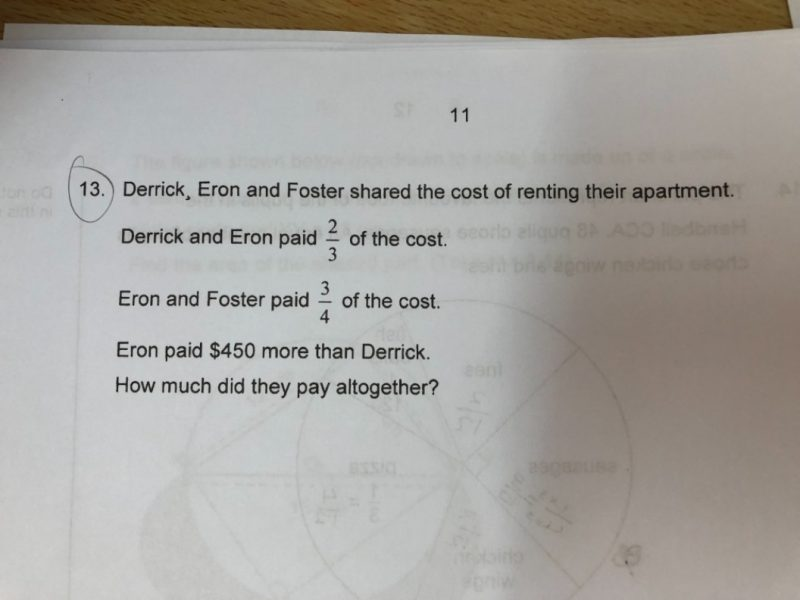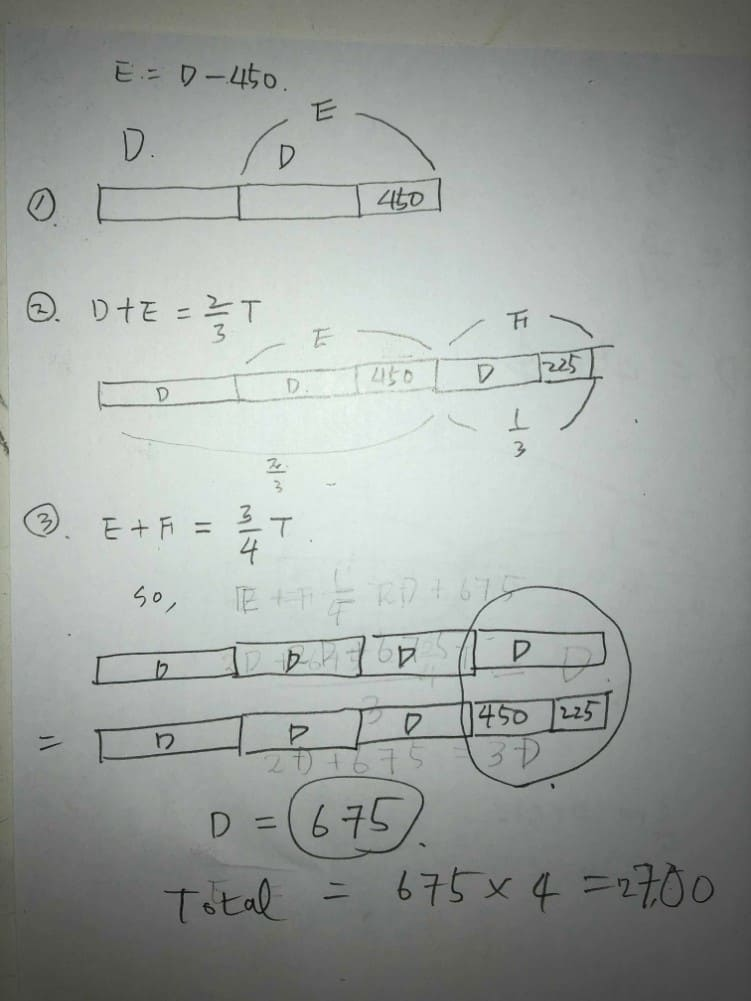# QuestionHi Shifus, appreciate your help with this question. Thank you in advance.

Source: Haig Girls’

I think this is easier to understand.

D           E            F        Total
2                  1             3
1                   3                     4
3u         5u          4u         12u

5u – 3u = 450
2u = 450
12u = 6 x 450 = 2700

Thank u THW 🙏

0 Replies 0 Likes

Derrick + Eron ——-> 2/3, Foster ——-> 1/3 = 4/12

Eron + Foster ——-> 3/4, Derrick ——-> 1/4 = 3/12

Eron ——-> 1 – (1/3) – (1/4) = 5/12

5 – 3 = 2

(450/2) x 12 = 2700

Ans : \$2700.

Thank you alfretztay

You are welcome, altp10.

0 Replies 0 Likes

Eron paid \$450 more than Derrick

\$450÷2=\$225

So

Derrick+Eron     Foster

x+(225+225+x)     225+x

Derrick = 1unit

Eron+Foster = 3units

So

Derrick      Eron                  Foster

x                  (225+225+x)     225+x

1unit                       3 units

Therefore

3x —> 225+225+x+225+x

3x –> 675+2x

x=675

Total

x + 225 + 225 + x + 225 + x

= 675+225+225+675+225+675

=2700

They paid \$2700 in total